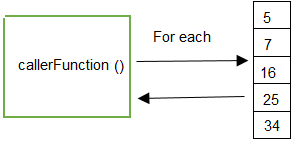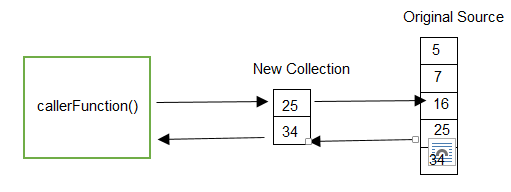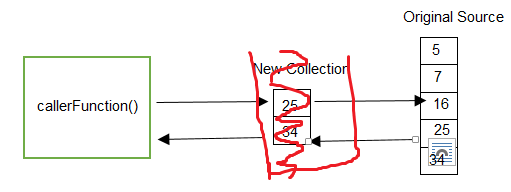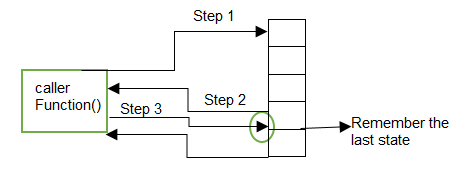© 2021 - First Crazy Developer (Abhishek Kumar)

###What is the use of "Yield" keyword in C#

Today we will  try to understand "What  exactly is the use of "Yield" keyword and what scenario we should use that?

"Yield" keywords helps to do custom stateful iteration over a collection. The main purpose of "Yield" keyword is Custom iteration & Stateful iteration.

Now we discuss these scenario with following code:-

` 1: public class YieldSample`
` 2:     {`
` 3:         public List<int> sampleList = new List<int>();`
` 4:  `
` 5:         public void setValue()`
` 6:         {`
` 7:             sampleList.Add(5);`
` 8:             sampleList.Add(7);`
` 9:             sampleList.Add(16);`
` 10:             sampleList.Add(25);`
` 11:             sampleList.Add(34);`
` 12:         }`
` 13:  `
` 14:         public void callerFunction()`
` 15:         {`
` 16:             setValue();`
` 17:             foreach (int temp in sampleList)`
` 18:             {`
` 19:                 Console.Write(temp);`
` 20:             }`
` 21:  `
` 22:         }`
` 23:     }`

In above code we declared variable "sampleList" which is list of integer types. Then defined a method named "setValue" . In this method we fill that list with some values. After that we defined a method named "callerFunction".In this method we are calling "setValue" method to fill the list and then what we are  doing through just  iterate over list help of foreach and print the value.

When we execute this program we got the output:-
5 7 16 25 34.

Above code is very simple program where we defined list then add value in that list then after we iterate over the list to print the value. Simple example over collection.

So now we visualise the above code model:So, let me do to make this scenario more complicated for better understanding, now we caller want to print the data which is greater than 20?

When this scenario comes into frame most developer change their code and write filter method to get the data.

Look the following code:

` 1: public class YieldSample`
` 2:     {`
` 3:         public List<int> sampleList = new List<int>();`
` 4:  `
` 5:         public void setValue()`
` 6:         {`
` 7:             sampleList.Add(5);`
` 8:             sampleList.Add(7);`
` 9:             sampleList.Add(16);`
` 10:             sampleList.Add(25);`
` 11:             sampleList.Add(34);`
` 12:         }`
` 13:  `
` 14:         public void callerFunction()`
` 15:         {`
` 16:             setValue();`
` 17:             foreach (int temp in FilterData())`
` 18:             {`
` 19:                 Console.Write(temp);`
` 20:             }`
` 21:  `
` 22:         }`
` 23:  `
` 24:         public IEnumerable<int> FilterData()`
` 25:         {`
` 26:             List<int> temp = new List<int>();`
` 27:             `
` 28:             foreach(int i in sampleList)`
` 29:             {`
` 30:                 if (i > 20)`
` 31:                 {`
` 32:                     temp.Add(i);`
` 33:                 }`
` 34:             }`
` 35:             return temp;`
` 36:         }`
` 37:     }`

In above code we add one method named "FilterData". In this we declare a temporary list variable, then we iterate  over sample list check the value is greater than 20, if value found add in temporary list after completion of iteration we return temporary list into caller function.

Now the output are:
25 34But when we go through the above code we found some issues like we declared a new list variable then add value into that and return that.What we need to do? Or think some other way....

Now we modified the above code:

` 1: public class YieldSample`
` 2:     {`
` 3:         public List<int> sampleList = new List<int>();`
` 4:  `
` 5:         public void setValue()`
` 6:         {`
` 7:             sampleList.Add(5);`
` 8:             sampleList.Add(7);`
` 9:             sampleList.Add(16);`
` 10:             sampleList.Add(25);`
` 11:             sampleList.Add(34);`
` 12:         }`
` 13:  `
` 14:         public void callerFunction()`
` 15:         {`
` 16:             setValue();`
` 17:             foreach (int temp in FilterData())`
` 18:             {`
` 19:                 Console.Write(temp);`
` 20:             }`
` 21:  `
` 22:         }`
` 23:  `
` 24:         public IEnumerable<int> FilterData()`
` 25:         {`
` 26:             foreach(int i in sampleList)`
` 27:             {`
` 28:                 if (i > 20)`
` 29:                 {`
` 30:                     yield return i;`
` 31:                 }`
` 32:             }`
` 33:             `
` 34:         }`
` 35:     }`

When we execute the above code we got same output:
25 34

Now we go to the internal process and know what happened in backend, when above  code executed. When we use the yield keyword, communication type is two way between caller and filter function. What is meaning of that?

When caller call the Filter function, iteration start on sample list and check the condition value is greater than 20, condition false(5>20) move the next value and again condition false (7>20) move the next value and again condition false(16>20), move the next value now condition is true, here is yield keyword so filter function back to caller function with that value and print that, then again caller function start communicating with filter function from last state here last state means loop iteration, again condition is true(34>20) and return to caller function and print that value.In above discussion I mentioned that when caller again back to filter function in that case yield maintain that state. To understand this concept we make the new scenario where we need to print total browsing list values.

Example: When I browse two list values 5 and 7 then output will be 12.

Look the following code:-

` 1: public class YieldSample`
` 2:     {`
` 3:         public List<int> sampleList = new List<int>();`
` 4:  `
` 5:         public void setValue()`
` 6:         {`
` 7:             sampleList.Add(5);`
` 8:             sampleList.Add(7);`
` 9:             sampleList.Add(16);`
` 10:             sampleList.Add(25);`
` 11:             sampleList.Add(34);`
` 12:         }`
` 13:  `
` 14:         public void callerFunction()`
` 15:         {`
` 16:             setValue();`
` 17:             foreach (int temp in AddTotal())`
` 18:             {`
` 19:                 Console.Write(temp);`
` 20:             }`
` 21:  `
` 22:         }`
` 23:  `
` 24:         public IEnumerable<int> AddTotal()`
` 25:         {`
` 26:             int total = 0;`
` 27:             foreach(int i in sampleList)`
` 28:             {`
` 29:                 yield return total=+i;`
` 30:                `
` 31:             }`
` 32:             `
` 33:         }`
` 34:     }`

When we execute the above code, the output:
5 12 28 53 87

In above code we create new method "AddTotal". In that we declare integer type variable total. In iteration block, we add browse value into total then use yield return.I already mentioned that when we use yield keyword, it maintain the last state,

so whenever caller function call "AddTotal", it start from last state, due to this reason total value is always maintained. In C# all collection are stateless, so if we need to maintain the state of collection we will use this keyword for that.

Now I hope we understood the use of "Yield" keyword in C#.Home Page 04 July 2015